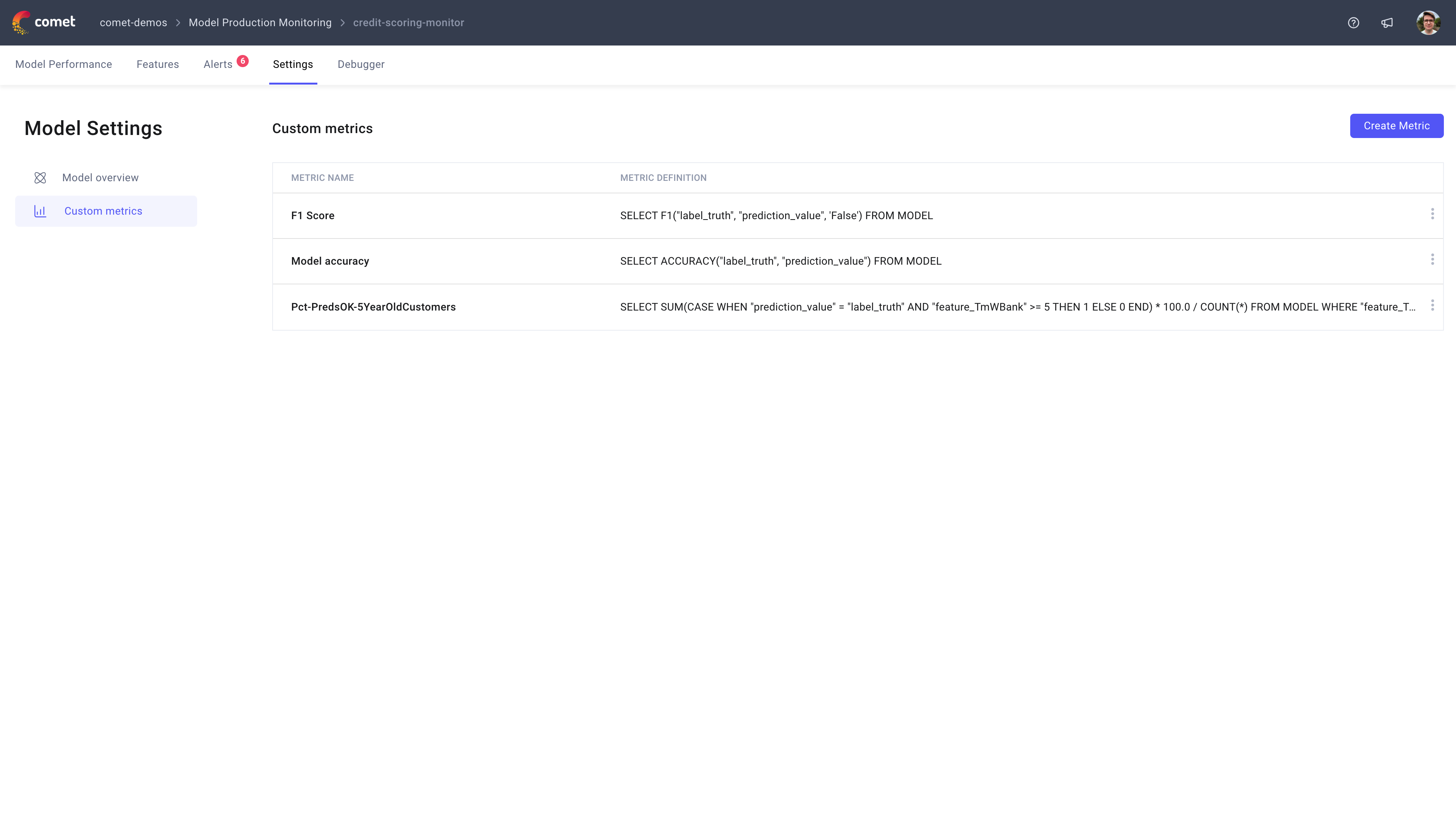# Custom metrics

Every machine learning model is unique and no monitoring solution is a one size fits all. MPM provides tracks the distribution of features, data drift and accuracy metrics out of the box but sometimes you just need more. This is where MPM Custom Metrics come into play.

## Custom Metric types¶

MPM Custom Metrics are broken down intro three metric types based on how they are computed:

• Confusion matrix: Custom metric defined based on the confusion matrix
• Numerical feature: Custom metric defined based on the quantile of a numerical feature
• Categorical feature: Custom metric defined based on the distribution of a categorical feature

For each of these metric types, MPM will compute the metrics in real-time depending on the filters you have selected (hourly vs daily data, specific model version, etc).

## Define a Custom Metric¶

Custom metrics are defined using the MPM settings tab under `Custom Metrics`.In order to define a metric you will need to specify a name for the metric, the metric type and the formula for the metric. The metric formula can be any mathematical expression.

The general concept behind the custom metrics feature is that there are a number of variables that you can use to define metrics. The value for these variables is automatically computed by MPM and differ based on the metric type:

• Confusion matrix: `confusion_matrix['<ground truth>']['<prediction>']`
• Numerical feature: `quantiles['<percentile>']`, the supported percentiles are `['p3', 'p6', 'p9', ..., 'p99']`
• Categorical: `values['<feature value>']`

Once a metric has been defined, it will appear as a new tab in the relevant section.## Common custom metrics¶

Here are some common custom metric formulas you can use to kick-start your metric include:

• Precision: `confusion_matrix['true']['true'] / (confusion_matrix['true']['true'] + confusion_matrix['true']['false'])`
• Recall: `confusion_matrix['true']['true'] / (confusion_matrix['true']['true'] + confusion_matrix['false']['true'])`
• p99: `quantiles['p99']`
• p99 deviation from baseline: `abs(quantiles['p99'] - 10)`
• Benelux country group: `values['france'] + values['belgium'] + values['luxembourg']`
Nov. 23, 2023